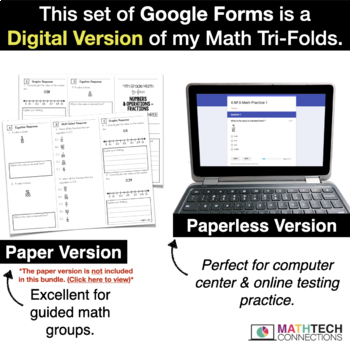digital

# 4th Grade Math Google FORMS - Fractions and Decimals: 21 Paperless ActivitiesSubject
Resource Type
Formats

TpT Digital Activity

PDF (9 MB)
Standards
\$9.50
TpT Digital Activity
Add notes & annotations through an interactive layer and assign to students via Google Classroom.
\$9.50
TpT Digital Activity
Add notes & annotations through an interactive layer and assign to students via Google Classroom.

#### Also included in

1. This 4th Grade Math Bundle includes 84 Google Forms Quizzes. These are PAPERLESS versions of my 4th Grade Math Tri-Folds.★ Included- 21 Quizzes: Fractions- 21 Quizzes: Measurement & Data- 9 Quizzes: Geometry- 18 Quizzes: Number & Operations in Base 10- 15 Quizzes: Operations & Algebraic
\$25.00
\$42.00
Save \$17.00
2. If you have access to Google Classroom, this Fourth Grade Digital Math Bundle will save you time and make math fun! This bundle includes math centers, spiral review, and assessments made specifically for use with Google Classroom.What's included?- Google Slides: Interactive slides that cover all fou
\$94.00
\$186.71
Save \$92.71
3. This 4th grade math bundle includes math tri-folds that are intended for use during small group instruction and Google Forms quizzes that can be used as pre and post math assessments. This 4th grade math practice bundle includes both PRINT and DIGITAL format. - The printable format includes 84 bookl
\$40.00
\$80.00
Save \$40.00

### Description

These Google Forms quizzes cover all 4th grade Fractions and Decimals standards. 21 math assessments aligned to the CCSS are included.

This digital resource is editable. You can edit/add/delete questions to fit your state's standards.

Standards/Topics Covered:

4.NF.1 - a total of 24 questions: I can use models to explain how multiplying the numerator and denominator of a fraction by the same number will not change the value of the fraction.

4.NF.2 - a total of 21 questions: I can compare two fractions with different numerators and different denominators by creating common denominators or numerators. I can use benchmark fractions when comparing fractions.

4.NF.3 - a total of 23 questions: I can understand addition and subtraction of fractions as joining and separating parts referring to the same whole. I can decompose a fraction into a sum of fractions with the same denominator. I can add and subtract mixed numbers with the same denominator.

4.NF.4 - a total of 27 questions: I can solve word problems involving multiplication of a fraction by a whole number. I can use visual fraction models and equations to represent the problem.

4.NF.5 - a total of 21 questions: I can change a fraction with denominator 10 to an equivalent fraction with denominator 100 in order to add the two fractions.

4.NF.6 - a total of 33 questions: I can use decimal notation for fractions with denominators 10 or 100.

4.NF.7 - a total of 30 questions: I can compare two decimals to the hundredths place by reasoning about their size. I can justify the comparisons by drawing a visual model.

1. Click on each link in the START HERE file

2. Create a copy of the Google Forms Quiz

4. Assign using Google Classroom or edit the settings so email isn't required

★ F.A.Q.

Yes. You will have to edit the settings so email isn't required. You then share the Google Forms link via your online platform (SeeSaw/TEAMS/etc)

Are these editable?

Yes.

The printable math tri-folds includes a Microsoft Word format.

The text in the Google Forms is editable. Images are not editable.

Can I use with Microsoft Teams/SeeSaw?

Yes.

You will have to edit the settings so email isn’t required.

You then share the link of the Google Form inside a Microsoft Teams or SeeSaw assignment.

*You will need a Google Drive account in order to save the form and then go back to it to check the grades.

Can I use with Schoology/Canvas/SeeSaw?

Yes.

In Schoology, you can go to Install App and select ‘Google Drive Resource App’.

★ Why Should you use Google Forms?

• Automatically Collects & Organizes Data
• Online Testing Practice with variety of question format types
• multiple choice
• multi-select
• table response
• drop down
• and more!

More PAPERLESS Resources

Total Pages
N/A
N/A
Teaching Duration
N/A
Report this Resource to TpT
Reported resources will be reviewed by our team. Report this resource to let us know if this resource violates TpT’s content guidelines.

### Standards

to see state-specific standards (only available in the US).
Compare two decimals to hundredths by reasoning about their size. Recognize that comparisons are valid only when the two decimals refer to the same whole. Record the results of comparisons with the symbols >, =, or <, and justify the conclusions, e.g., by using a visual model.
Use decimal notation for fractions with denominators 10 or 100. For example, rewrite 0.62 as 62/100; describe a length as 0.62 meters; locate 0.62 on a number line diagram.
Express a fraction with denominator 10 as an equivalent fraction with denominator 100, and use this technique to add two fractions with respective denominators 10 and 100. For example, express 3/10 as 30/100, and add 3/10 + 4/100 = 34/100.
Solve word problems involving multiplication of a fraction by a whole number, e.g., by using visual fraction models and equations to represent the problem. For example, if each person at a party will eat 3/8 of a pound of roast beef, and there will be 5 people at the party, how many pounds of roast beef will be needed? Between what two whole numbers does your answer lie?
Understand a multiple of 𝘢/𝘣 as a multiple of 1/𝘣, and use this understanding to multiply a fraction by a whole number. For example, use a visual fraction model to express 3 × (2/5) as 6 × (1/5), recognizing this product as 6/5. (In general, 𝘯 × (𝘢/𝘣) = (𝘯 × 𝘢)/𝘣.)